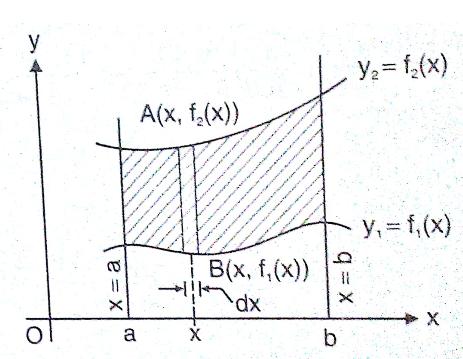# The area (in square units) of the region bounded by the curves y + 2x2 = 0 and y + 3x2 = 1 , is equal to : Option 1)Option 2)Option 3)Option 4)As learnt in concept

Area along x axis -

Letbe two curve then area bounded between the curves and the lines

x = a and x = b is- whereinWherey+2x2=0; y+3x2=1

y= -2x2; y=1-3x2

Calculate the point of intersection first

-2x2= 1-3x2

=> x2=1

=> x=1=>=>Option 1)this is incorrect option

Option 2)this is incorrect option

Option 3)this is incorrect option

Option 4)this is correct option